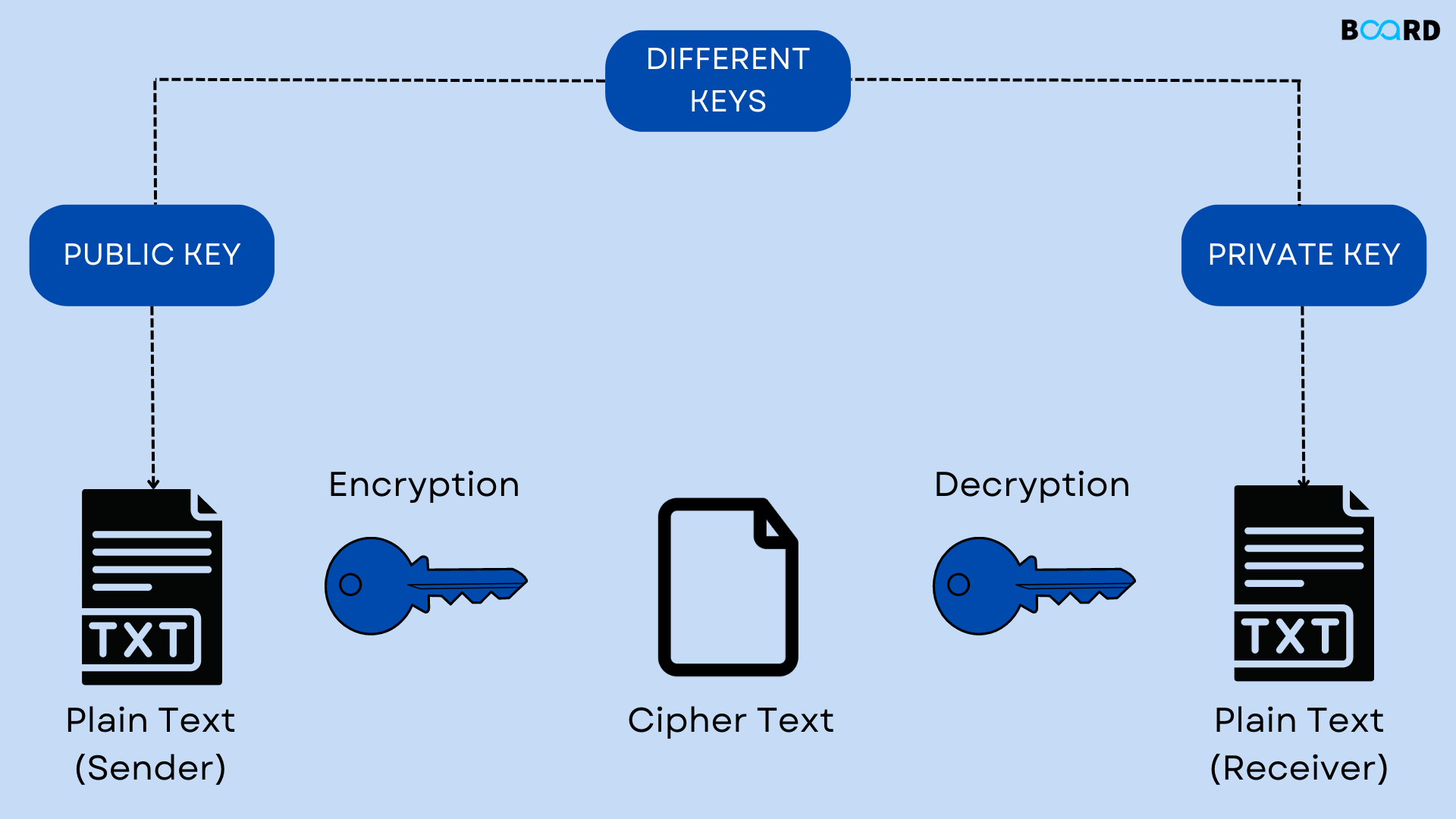# Cryptography: Explanation and Types## Introduction

It is the study of secure communication within networks. It is a technique where only the recipient and sender can understand the message. The term is derived from prefixes i.e, “crypt” which means hidden, and the suffix “graphy” which indicates writing. Many mathematical computations and sets of rules included in calculations are used for encrypting the data which has to be sent to the receiver.

## Techniques used for Cryptography

Techniques of cryptography include converting the data into the encrypted code called a cipher which has to be sent to the receiver and this process is known as encryption. Once the data is transferred to the receiver the cipher text is converted into normal text/plain text, this procedure is called decryption.

## Types of Cryptography

Based on the techniques of cryptography are divided into three major types which are discussed clearly in the below article:

### Symmetric Key Cryptography

In this type of cryptography, the encryption technique is where the receiver and sender have the same common key. The main drawback of this type of cryptography is the receiver and sender have to find a way to exchange the key in such a manner that it is secured. A common example of Symmetric key cryptography is Data Encryption System (DES).

### Hash Functions

No key is used in this type of cryptography. A hash value is calculated with a fixed length as per the plain text which makes it impossible for the contents of plain text to be recovered. The common example of Hash Function type cryptography is many operating systems use hash functions to encrypt passwords.

### Asymmetric Key Cryptography

Two keys are used in this type of asymmetric key cryptography. There are two types of keys which are public, and private keys. The public key is used for encryption and the private key is used for decryption. The public key is known to everyone but is encoded only with the help of the private key present at the receiver end side.

## Applications of Cryptography

• Transaction through Digital Currency